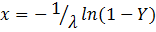Generate Random Numbers

A common requirement is to generate a set of random numbers that meet some underlying criterion.  For example, a set of numbers that are uniformly distributed from 1 to 100.  Alternatively, one might want random numbers from some other distribution such as a standard normal distribution.

While there are specialized algorithms to generate random numbers from specific distributions, a common approach relies on generating uniform random numbers and then using the inverse function of the desired distribution.  For example, to generate a random number from a standard normal distribution, use =NORM.S.INV(RAND())

Another common requirement is the generation of integer random numbers from a uniform distribution.  This might be to select people for something like, say, training, or a drug test.  Or, it might be to pick a winner for a door prize at a social event.  It might also be to assign players to groups for a sport tournament such as golf.

Given the range of applications that call for uniform random numbers, this note starts with that topic.

Uniform real random numbers

The easiest way to generate uniform random numbers is to use the RAND function.  According to a Microsoft web post, the current version of RAND uses a much improved algorithm. In a separate blog post, Microsoft notes that it has improved several functions with Excel 2010.  It generates a uniform random number that is greater than or equal to 0 and less than 1, i.e., the generated random number X satisfies the rule 0 <= X < 1.

We can extend the value of the RAND function to a larger range by simply multiplying the function value by the desired range.  So, to generate a random number Y that satisfies 0 <= Y < 100, use =100*RAND()

We can shift the number range by adding the desired lowest value.  So, to generate uniform random numbers in the range of -5 <= Z < +5, use the formula =-5 + 10*RAND().

Uniform integer random numbers

The easiest way to generate uniform integer random numbers is to convert the above real random numbers to integers.  And, that is easy with Excel’s TRUNC function.

To generate integer random numbers between 1 and 10, take the integer portion of the result of real uniform numbers between that are <=1 and <11.  The reason this truncation works is that given uniform real random numbers 1 <= X < 11, the truncated result will be integers between 1 and 10, each with equal probability.

So, to generate uniform integer random numbers between M and N, where M < N, use =M+INT(RAND()*(N-M+1))

One common mistake

A significant mistake, and one that I have found even in published books, is the use of the ROUND function to generate integer uniform random numbers as in =ROUND(RAND()*2,0) to generate random numbers in the set {0, 1, 2}.  This is simply wrong.  The boundary values will have a probability that is ½ the probability of the other values.  There are ways to use ROUND to generate random integers that follow a uniform distribution but since INT is so easy to use, why bother with ROUND?

Random numbers from other distributions

One of the useful properties of generating numbers from some probability distribution is that the CDF (cumulative distribution function) itself follows a uniform distribution between 0 and 1.

So, we can generate random numbers from any distribution for which Excel supports an inverse of the CDF (or for which we can write the inverse of the CDF) or for any distribution for which we can write a formula for the inverse of the CDF.

Since Excel contains a built-in function for the inverse of the standard normal distribution, we can generate random numbers that follow the standard normal random with the formula =NORM.S.INV(RAND()).  The same technique works for other distributions for which Excel supports the inverse function.

In those cases where Excel does not include an inverse distribution function, such as for an exponential distribution, one may still be able to create the inverse function.  For example, the exponential CDF is given by, where λ is the exponential distribution parameter.  That gives us the inverse as.  So, with λ in cell N4, the formula =-1/\$N\$4*LN(1-RAND()) yields a random number from the exponential distribution with parameter λ.  We can use this technique for any distribution for which we can write the formula for the inverse of the CDF.

 Description of the RAND function in Excel (http://support.microsoft.com/kb/828795)

 Function Improvements in Excel 2010
(http://blogs.office.com/b/microsoft-excel/archive/2009/09/10/function-improvements-in-excel-2010.aspx)